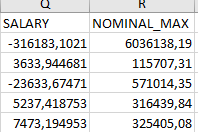# SQL Adding a minus in a number if it is in another

I have a question for you, I don't know how to do it, I need to create a procedure if or case when. Relying on the back in the Salary column, some value has a minus, e.g. -316183.1021. This will add a minus to the value in the NOMINAL MAX column
For example here: ( This is result after sql in excel )``````    If SALARY = "-" then NOMINAL_MAX*(-1)```
``````

if Salary<0 then nominal_max*-1

This /* I added "This" solely because the site wouldn't accept only the code below as a valid post */

SIGN(SALARY) * NOMINAL_MAX

1 Like